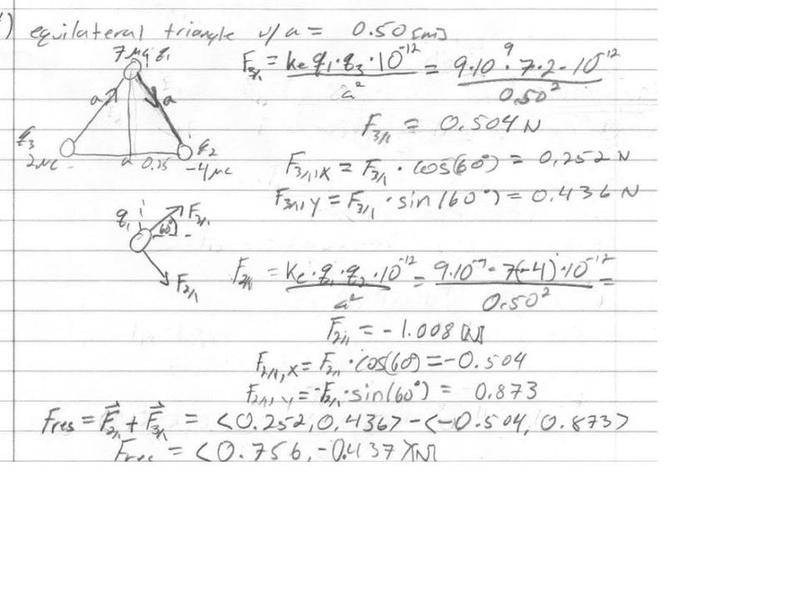# Forces between charges. Did the problem, have question on the result.

## Homework Statement

Three point charges are located at the corners of an equilateral triangle with side length a=0.50m

## The Attempt at a Solution

My question is how can I subtract the vector F2/1? Vectors cant be negative right? I found the magnitude of the vector to be negative. Should I have not included the negative sign for the 4 micro coulomb charge? Then I add the two vectors and that will give me the correct result.

My result is correct, but I cant minus vectors so I kind of did it illegally ya?Why do you think that vectors cant be negative? You can subtract vectors with no problem, whoever told you this was wrong. A negative vector is an arrow pointing in the opposite direction of whatever the unit vector is. basically the vector itself isnt negative, but the magnitude is negative. for example if I have a vector 3x (3 in the x direction) it represents an arrow of magnitude 3 pointing in the x direction. --->. A vector -3x (-3 in the x direction) just represents an arrow of magnitue -3 in the x direction. <---. Don't get confused by the term "vector addition". When we subtract, all we are really doing is adding a negative number. Subtracting vectors is solely adding vectors of negative magnitude. Which is totally fine.

Why do you think that vectors cant be negative? You can subtract vectors with no problem, whoever told you this was wrong. A negative vector is an arrow pointing in the opposite direction of whatever the unit vector is. basically the vector itself isnt negative, but the magnitude is negative. for example if I have a vector 3x (3 in the x direction) it represents an arrow of magnitude 3 pointing in the x direction. --->. A vector -3x (-3 in the x direction) just represents an arrow of magnitue -3 in the x direction. <---. Don't get confused by the term "vector addition". When we subtract, all we are really doing is adding a negative number. Subtracting vectors is solely adding vectors of negative magnitude. Which is totally fine.

Ok, that sounds very familiar to how I studied it in math.

I dont know why I can get confused with vectors.. I think its because my intro to physics teacher said that only magnitudes (scalars) are positive. If your vector comes up negative, you have initially set it in the wrong direction.

I know part of that is true, or maybe all if it in a way, I just interpreted the first part wrong I think..

So the work I wrote is correct? By adding the vectors, I subtract the components of F2/1 from F3/1

Yeah it looks fine to me, i don't have time to look into detail. You should talk to your physics teacher and tell him that the way he is saying it is confusing. I am sure he will appreciate feedback on his teaching.